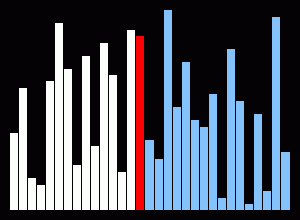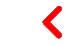# 15 Sorting Algorithms in 6 Minutes

+1 Likes (1)

## Sorting Algorithmssorting

Visualization and “audibilization” of 15 Sorting Algorithms in 6 Minutes.
Sorts random shuffles of integers, with both speed and the number of items adapted to each algorithm’s complexity.

The algorithms are:

1. selection sort
1. Selection sort is an in-place comparison sort. It has O(n2) complexity, making it inefficient on large lists, and generally performs worse than the similar insertion sort. Selection sort is noted for its simplicity, and also has performance advantages over more complicated algorithms in certain situations. The algorithm finds the minimum value, swaps it with the value in the first position, and repeats these steps for the remainder of the list. It does no more than n swaps, and thus is useful where swapping is very expensive.
2. insertion sort
1. Insertion sort is a simple sorting algorithm that is relatively efficient for small lists and mostly sorted lists, and is often used as part of more sophisticated algorithms. It works by taking elements from the list one by one and inserting them in their correct position into a new sorted list. In arrays, the new list and the remaining elements can share the array’s space, but insertion is expensive, requiring shifting all following elements over by one. Shell sort (see below) is a variant of insertion sort that is more efficient for larger lists.
3. quick sort
1. Quicksort is a divide and conquer algorithm which relies on a partition operation: to partition an array an element called a pivot is selected. All elements smaller than the pivot are moved before it and all greater elements are moved after it. This can be done efficiently in linear time and in-place. The lesser and greater sublists are then recursively sorted. This yields average time complexity of O(n log n), with low overhead, and thus this is a popular algorithm. Efficient implementations of quicksort (with in-place partitioning) are typically unstable sorts and somewhat complex, but are among the fastest sorting algorithms in practice. Together with its modest O(log n) space usage, quicksort is one of the most popular sorting algorithms and is available in many standard programming libraries.
4. merge sort
1. Merge sort takes advantage of the ease of merging already sorted lists into a new sorted list. It starts by comparing every two elements (i.e., 1 with 2, then 3 with 4…) and swapping them if the first should come after the second. It then merges each of the resulting lists of two into lists of four, then merges those lists of four, and so on; until at last two lists are merged into the final sorted list. Of the algorithms described here, this is the first that scales well to very large lists, because its worst-case running time is O(n log n). It is also easily applied to lists, not only arrays, as it only requires sequential access, not random access. However, it has additional O(n) space complexity, and involves a large number of copies in simple implementations.
5. heap sort
1. Heapsort is a much more efficient version of selection sort. It also works by determining the largest (or smallest) element of the list, placing that at the end (or beginning) of the list, then continuing with the rest of the list, but accomplishes this task efficiently by using a data structure called a heap, a special type of binary tree. Once the data list has been made into a heap, the root node is guaranteed to be the largest (or smallest) element. When it is removed and placed at the end of the list, the heap is rearranged so the largest element remaining moves to the root. Using the heap, finding the next largest element takes O(log n) time, instead of O(n) for a linear scan as in simple selection sort. This allows Heapsort to run in O(n log n) time, and this is also the worst case complexity.
1. Radix sort is an algorithm that sorts numbers by processing individual digits. n numbers consisting of k digits each are sorted in O(n · k) time. Radix sort can process digits of each number either starting from the least significant digit (LSD) or starting from the most significant digit (MSD). The LSD algorithm first sorts the list by the least significant digit while preserving their relative order using a stable sort. Then it sorts them by the next digit, and so on from the least significant to the most significant, ending up with a sorted list.
1. While the LSD radix sort requires the use of a stable sort, the MSD radix sort algorithm does not (unless stable sorting is desired). In-place MSD radix sort is not stable. It is common for the counting sort algorithm to be used internally by the radix sort. A hybrid sorting approach, such as using insertion sort for small bins improves performance of radix sort significantly.
8. std::sort (intro sort)
10. shell sort
1. Shell sort was invented by Donald Shell in 1959. It improves upon bubble sort and insertion sort by moving out of order elements more than one position at a time. One implementation can be described as arranging the data sequence in a two-dimensional array and then sorting the columns of the array using insertion sort.
11. bubble sort
1. Bubble sort is a simple sorting algorithm. The algorithm starts at the beginning of the data set. It compares the first two elements, and if the first is greater than the second, it swaps them. It continues doing this for each pair of adjacent elements to the end of the data set. It then starts again with the first two elements, repeating until no swaps have occurred on the last pass. This algorithm’s average time and worst-case performance is O(n2), so it is rarely used to sort large, unordered data sets. Bubble sort can be used to sort a small number of items (where its asymptotic inefficiency is not a high penalty). Bubble sort can also be used efficiently on a list of any length that is nearly sorted (that is, the elements are not significantly out of place). For example, if any number of elements are out of place by only one position (e.g. 0123546789 and 1032547698), bubble sort’s exchange will get them in order on the first pass, the second pass will find all elements in order, so the sort will take only 2n time.
12. cocktail shaker sort
1. ocktail shaker sort, also known as bidirectional bubble sort, cocktail sort, martini sort, shaker sort (which can also refer to a variant of selection sort), ripple sort, shuffle sort, or shuttle sort, is a variation of bubble sort that is both a stable sorting algorithm and a comparison sort. The algorithm differs from a bubble sort in that it sorts in both directions on each pass through the list. This sorting algorithm is only marginally more difficult to implement than a bubble sort, and solves the problem of turtles in bubble sorts. It provides only marginal performance improvements, and does not improve asymptotic performance; like the bubble sort, it is not of practical interest (insertion sort is preferred for simple sorts), though it finds some use in education.
13. gnome sort
1. Gnome sort (or Stupid sort) is a sorting algorithm originally proposed by Dr. Hamid Sarbazi-Azad (Professor of Computer Engineering at Sharif University of Technology) in 2000 and called “stupid sort” (not to be confused with bogosort), and then later on described by Dick Grune and named “gnome sort”. It is a sorting algorithm which is similar to insertion sort, except that moving an element to its proper place is accomplished by a series of swaps, as in bubble sort. It is conceptually simple, requiring no nested loops. The average, or expected, running time is O(n2), but tends towards O(n) if the list is initially almost sorted.
14. bitonic sort
1. Bitonic mergesort is a parallel algorithm for sorting. It is also used as a construction method for building a sorting network. The algorithm was devised by Ken Batcher. The resulting sorting networks consist of O ( n log ⁡ ( n ) ^ 2 ) comparators and have a delay of O ( log ⁡ ( n ) ^ 2 ) , where “n” is the number of items to be sorted.
15. bogo sort (30 seconds of it).
1. In computer science, bogosort (also permutation sort, stupid sort, slowsort, shotgun sort or monkey sort) is a particularly ineffective sorting algorithm based on the generate and test paradigm. The algorithm successively generates permutations of its input until it finds one that is sorted. It is not useful for sorting, but may be used for educational purposes, to contrast it with other more realistic algorithms.
Follow: